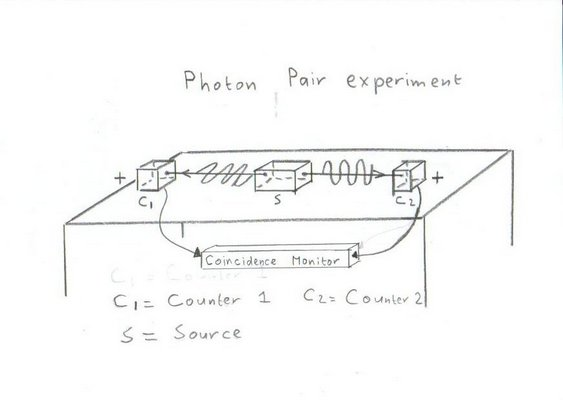## Experiment 1 with Entangled photonsThe assumption is:
• That the left photon moves towards the left and is polarised in the horizontal plane .
• That the right photon moves towards the right and is also polarised in the horizontal plane .
The idea behind this experiment is to test the quality of the source in generating photon pairs with the aid of a Coincidence Monitor.

The result of the test will be 2 sequence of events, showing the arrival times of the photons.

```Counter 1: x x  x x  x x x  x  x x   x  x   x x    x  x x   x tn1 = 18
Counter 2: x x  x    x x    x  x x   x  x x x x  x x  x x   x tn2 = 18
```
The above pattern shows that in total 20 photon pairs are created, but that there are only 18 are counted (at each side), meaning 2 are missing.

This type of error should be included if more is added to this experiment and filters are added.